Next: Register Combiners Up: Parameter Interpolation and Normalization Previous: Spherical Interpolation

### Cube-Map

One method to renormalize the vectors is to use a cube map texture. Cube map texturing is a form of texture mapping that uses a 3D direction vector built from the texture coordinates (s, t, r) to access a texture that consists of six square 2D images arranged like the faces of a cube .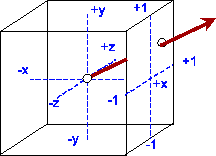[Directional Access] ]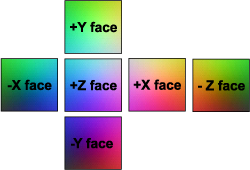[RGB Coded Normalization Faces
In order to normalize a vector, the cube map can be thought as a way to store a look-up table indexed by a direction vector (Figure 5(a)). This means that vectors of varying length which point in the same direction do not change the lookup result. The normalization given in Formula 4 is precomputed for discrete values of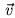and stored in the cube map.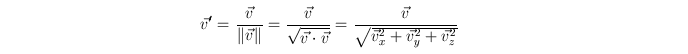(4)

Since parts of the render pipeline work only with positive values, the signed vector components must be range compressed from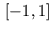to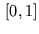and stored as colors (Figure 5(b)).Next: Register Combiners Up: Parameter Interpolation and Normalization Previous: Spherical Interpolation
Gerald Schröcker 2002-03-21